## Posts Tagged ‘POV-Ray

10
Feb
09

### Scherk-Collins SurfaceThis surface can be formed by twisting and warping a singly-periodic Scherk’s minimal surface. This idea was originally attributed to Brent Collins. Technically, the surface is no longer considered exactly “minimal” after twisting but it still looks minimal (it is actually very difficult to find the exact shape for most minimal surfaces). Click here to download some POV-Ray code.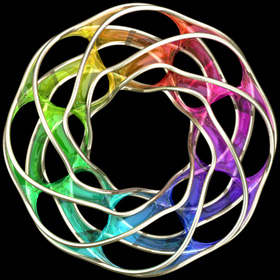Here is some Mathematica code:
```(* runtime: 0.3 second *) << Graphics`Master`; n = 5; r = 0.75n; Twist[{x_, y_, z_}, theta_] := {x Cos[theta] - y Sin[theta], x Sin[theta] + y Cos[theta], z}; Warp[{x_, y_, z_}, theta_] := {(x + r) Cos[theta], (x + r) Sin[theta], y}; f[z_] := Module[{t1 = Sqrt[2Cot[z]], t2 = Cot[z] + 1}, Warp[Twist[Re[{0.5xsign(Log[t1 - t2] - Log[t1 + t2])/Sqrt, ysign I(ArcTan[1 - t1] - ArcTan[1 + t1])/Sqrt, z}], 2Re[z]/n], 2Re[z]/n]]; DisplayTogether[Table[ParametricPlot3D[f[x + I y], {x, 0, n Pi}, {y, 0.001, 0.75}, PlotPoints -> {8n + 1, 5}, Compiled -> False], {xsign, -1, 1, 2}, {ysign, -1, 1, 2}]]```

The following Mathematica code can be used to increase the number of edges (or “branches”). This code uses some complicated functions that were adapted from Matthias Weber’s Mathematica notebook:
```(* runtime: 1.2 seconds *) << Graphics`Shapes`; k = 4; phi = Pi(0.6/k - 0.5)/(1 - k); f[z_] := Re[NIntegrate[Evaluate[{0.5 (w^(1 - k) - w^(k - 1)), 0.5 I (w^(1 - k) + w^(k - 1)), 1}/(w^(k + 1) + w^(1 - k) - 2w Cos[k phi])], {w, 0, z}]]; alpha = Pi/k; zbeta = Exp[I Pi(phi/alpha - 0.5)]; surface = ParametricPlot3D[Re[f[Exp[I alpha/2]((1 + I zbeta Exp[r + I theta])/(I Exp[r + I theta] -zbeta))^(alpha/Pi)]], {r, 0, 4}, {theta, 0, Pi}, PlotPoints -> 10, Compiled -> False, DisplayFunction -> Identity][]; z0 = f[]; surface = {surface, AffineShape[TranslateShape[surface, {0, 0, -2z0}], {1, 1, -1}]}; surface = {surface, AffineShape[surface, {1, -1, 1}]}; surface = Table[RotateShape[surface, 2Pi i/k, 0, 0], {i, 1, k}]; dz = Pi Csc[k phi]/k; Show[Graphics3D[Table[TranslateShape[surface, {0, 0, i dz}], {i, 0, 1}]]] ```

21
Jan
09

### Punctured Helicoid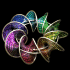Here is a helicoidwith holes in it. The following Mathematica code uses some complicated functions that were adapted from Matthias Weber’sMathematica notebook:
```(* runtime: 4 seconds *) << Graphics`Shapes`; tau0 = Exp[1.23409 I]; b0 = 0.629065; theta[z_] := EllipticTheta[1, Pi z, Exp[I Pi tau0]]; r1[z_] := theta[z + 0.5 (b0 - 2) (tau0 + 1)]/theta[z + 0.5 (b0 - 1) (tau0 + 1)]; r2[z_] := theta[z - 0.5 b0 (tau0 + 1)]/theta[z - 0.5 (b0 + 1) (tau0 + 1)]; omega3[z_] := r1[z] r2[z]/(0.386191 - 0.169839 I); G[z_] := (108.37 - 62.8417 I) Exp[I Pi (b0 - 2 z + 2 tau0 + b0 tau0)]r1[z]/r2[z]; f[z0_] := Re[NIntegrate[Evaluate[{-(G[z] omega3[z] - omega3[z]/G[z] )/2, I(G[z] omega3[z] + omega3[z]/G[z] )/2, omega3[z]}], {z, tau0/2, z0}]] + {0.434156, 0, -1}; a0 = -0.409956; r0 = 2.43051; g[z_] := (EllipticF[ArcSin[(a0 + r0 E^z)/(1 - a0 E^z)], 1/r0^2]/(2EllipticF[Pi/2, 1/r0^2]) + 0.5)(1 + tau0)/2; surface = ParametricPlot3D[f[g[x + I y]], {x, -2.5, 2.5 - 0.8881}, {y, 0.001,0.999Pi}, PlotPoints -> {15, 10}, Compiled -> False, DisplayFunction -> Identity][]; surface = {surface, RotateShape[surface, 0, 0, Pi]}; Show[Graphics3D[{surface, TranslateShape[surface, {0, 0, 2}]}, ViewPoint -> {1, 6, 3}]];```

21
Oct
08

### Dodecaplex (120 Cell)Polychorons are the 4D version of polyhedrons. One way to visualize a polychoron is to apply a 4D to 3D stereographic projection to it. A dodecaplex is a uniform 4D polychoron composed 120 dodecahedral cells. These cells can be divided into 12 rings (Hopf fibrations) of 10 cells each. This picture shows a stereographic projection of 6 rings of the dodecaplex. Each ring is shown in a different color, but only 5 rings are open to direct view because they are wrapped around the 6th ring. I first saw this concept on Matthias Weber’s book page. Click here to download some POV-Ray code.10
Oct
08

### Stereographic Projection of a Dodecahedron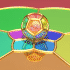Here is a stereographic projection of a dodecahedron. This is the 3D counterpart to the 4D dodecaplex. Here is some Mathematica code:
```(* runtime: 0.4 second *) z1 = (Sqrt - 3)/Sqrt[30.0 - 6 Sqrt]; z2 = Sqrt[(1 + 2/Sqrt)/3.0]; r1 = Sqrt[2(1 + 1/Sqrt)/3.0]; r2 = Sqrt[2(1 - 1/Sqrt)/3.0]; vertices = Join[Table[{r2 Cos[theta], r2 Sin[theta], z2}, {theta, 0, 2Pi - 0.4Pi, 0.4Pi}], Table[z1 = -z1; {r1 Cos[theta], r1 Sin[theta], z1}, {theta, 0, 1.8Pi, 0.2Pi}], Table[{r2 Cos[theta], r2 Sin[theta], -z2}, {theta, 0.2Pi, 1.8Pi, 0.4Pi}]]; edges = {{1, 2}, {2, 3}, {3, 4}, {4, 5}, {5, 1}, {1, 6}, {2, 8}, {3, 10}, {4, 12}, {5, 14}, {6, 7}, {7, 8}, {8, 9}, {9, 10}, {10, 11}, {11, 12}, {12, 13}, {13, 14}, {14, 15}, {15, 6}, {7, 16}, {9, 17}, {11, 18}, {13, 19}, {15, 20}, {16,17}, {17, 18}, {18, 19}, {19, 20}, {20, 16}}; Show[Graphics3D[Map[Line[vertices[[#]]] &, edges]]] Norm[x_] := x.x; Normalize[x_] := x/Sqrt[x.x]; Rx[theta_] := {{1, 0, 0}, {0, Cos[theta], -Sin[theta]}, {0,Sin[theta], Cos[theta]}}; ProjectPoint[{x_, y_, z_}] := 2{x, y}/(1 - z); ProjectSegment[{v1_, v2_}] := Module[{p1 = ProjectPoint[v1], p2 = ProjectPoint[v2]}, {nx, ny, nz} = Normalize[Cross[v1, v2]]; If[nz != 0, p0 = -2{nx, ny}/nz; r = 2/Abs[nz]; theta = Sign[nz]Re[ArcCos[(p1 - p0).(p2 - p0)/Sqrt[Norm[p1 - p0]Norm[p2 - p0]]]], theta = 0]; If[Abs[theta] > 0.001, theta1 = ArcTan[p1[] - p0[], p1[] - p0[]]; theta2 = theta1 + theta; If[theta1 > theta2, t = theta1; theta1 = theta2; theta2 = t]; Circle[p0, r, {theta1, theta2}], Line[{p1, p2}]]]; Do[Show[Graphics[Map[ProjectSegment[Map[Rx[phi].# &, vertices[[#]]]] &, edges], PlotRange -> 6{{-1, 1}, {-1, 1}}, AspectRatio -> Automatic]], {phi, 0, 2Pi, Pi/18}];```

01
Oct
08

### Double SpiralOne way to create a Double Spiralis by applying a light projection from the top of a Riemann sphere (loxodrome) onto a plane.

This type of projection is called a stereographic projection.

```(* runtime: 3 seconds *) << Graphics`Shapes`; a = 0.25; Rx[phi_] := {{1, 0, 0}, {0, Cos[phi], -Sin[phi]}, {0, Sin[phi], Cos[phi]}}; Do[loxodrome = Table[Rx[phi].{Sin[t], -a t, -Cos[t]}/Sqrt[1 + (a t)^2], {t, -100, 100, 0.1}]; projection = Map[Module[{r = 2/(1 - #[])}, {r #[],r #[], -1}] &, loxodrome]; Show[Graphics3D[{EdgeForm[], Sphere[0.99, 37, 19], Polygon[{{4, 4, -1}, {-4, 4, -1}, {-4, -4, -1}, {4, -4, -1}}],Line[loxodrome], Line[projection]},PlotRange -> {{-4, 4}, {-4, 4}, {-1, 1}}]], {phi, 0, Pi -Pi/12, Pi/12}] ```
Another kind of double spiral can be made by applying a special homography to a single logarithmic spiral:
```(* runtime: 0.05 second *) Show[Graphics[Table[Line[Table[z = Exp[r + (2 r + theta)I]; z = (1 + z)/(1 - z); {Re[z], Im[z]}, {r, -10, 10, 0.1}]], {theta, -Pi, Pi, Pi/3}], PlotRange -> {{-2, 2}, {-2, 2}}, AspectRatio -> Automatic]]```Here is some Mathematica code that uses the inverse method:
```(* runtime: 17 seconds *) Show[Graphics[RasterArray[Table[r1 = (x - 1)^2 + y^2; r2 = (x + 1)^2 + y^2; Hue[(Sign[y]ArcCos[(x^2 + y^2 - 1)/Sqrt[r1 r2]] -Log[r1/r2])/(2Pi)], {x, -2, 2, 4/274}, {y, -2, 2, 4/274}]], AspectRatio -> 1]]```
and here is some POV-Ray code:
```// runtime: 2 seconds camera{orthographic location <0,0,-2> look_at 0 angle 90} #declare r1=function(x,y) {(x-1)*(x-1)+y*y}; #declare r2=function(x,y) {(x+1)*(x+1)+y*y}; #declare f=function{(y/abs(y)*acos((x*x+y*y-1)/sqrt(r1(x,y)*r2(x,y)))-ln(r1(x,y)/r2(x,y)))/(2*pi)}; plane{z,0 pigment{function{f(x,y,0)}} finish{ambient 1}}```

25
Aug
08

### Cross Section of the Quintic Calabi-Yau ManifoldThe left picture shows a 3D cross section of the quintic 6D Calabi-Yau Manifold proposed for String Theory. The right picture shows various degrees of complexity.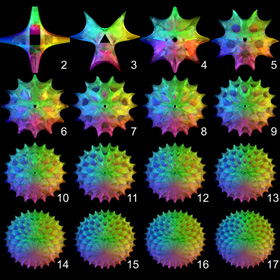Here is some Mathematica code:
```(* runtime: 54 seconds, change n for other degrees of complexity *) n = 5; CalabiYau[z_, k1_, k2_] := Module[{z1 = Exp[2Pi I k1/n]Cosh[z]^(2/n), z2 = Exp[2Pi I k2/n]Sinh[z]^(2/n)}, {Re[z1], Re[z2], Cos[alpha]Im[z1] + Sin[alpha]Im[z2]}]; Do[alpha = (0.25 + t)Pi; Show[Graphics3D[Table[ParametricPlot3D[CalabiYau[x + I y, k1, k2], {x, -1, 1}, {y, 0, Pi/2}, DisplayFunction -> Identity, Compiled ->False][], {k1, 0, n - 1}, {k2, 0, n - 1}], PlotRange -> 1.5{{-1, 1}, {-1, 1}, {-1, 1}}, ViewPoint -> {1, 1, 0}]], {t, 0, 1, 0.1}];```

12
Aug
08

### Clifford Torus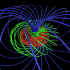The Hopf map is a special transformation invented by Heinz Hopf that maps to each point on the ordinary 3D sphere from a unique circle of points on the 4D sphere. Taken together, these circles form a fiber bundle called a Hopf Fibration. If you apply a 4D to 3D stereographic projection to the Hopf Fibration, you get a beautiful 3D torus called a Clifford Torus composed of interlinked Villarceau circles.

By applying 4D rotations to the Hopf Fibration, you can transform the Clifford Torus into a Dupin cyclide or you can turn it inside-out.

Here is some Mathematica code:
```(* runtime: 7 seconds *) HopfInverse[theta_, phi_, psi_] := {Cos[phi/2] Cos[psi], Cos[phi/2]Sin[psi], Cos[theta + psi]Sin[phi/2], Sin[theta + psi]Sin[phi/2]}; Ryw[theta_] := {{1, 0, 0, 0}, {0, Cos[theta], 0, Sin[theta]}, {0, 0, 1, 0}, {0, -Sin[theta], 0, Cos[theta]}}; StereographicProjection[{x_, y_, z_, w_}] := {x, y, z}/(1 - w); Table[Show[Graphics3D[Table[{Hue[(4 phi/Pi - 1)/3], Table[Line[Table[StereographicProjection[Ryw[alpha].HopfInverse[theta, phi, psi]], {psi, 0.0, 2Pi, Pi/18}]], {theta, 0.0, 2 Pi,Pi/9}]}, {phi, Pi/4, 3Pi/4, Pi/4}], PlotRange -> 3{{-1, 1}, {-1, 1}, {-1, 1}}]], {alpha, 0, Pi, Pi/18}];```

## Welcome !

You will find here some of my favorite hobbies and interests, especially science and art.

I hope you enjoy it!

Subscribe to theRSS feed to stay informed when I publish something new here.

I would love to hear from you! Please feel free to send me an email : bugman123-at-gmail-dot-com

## TopicsBerna Blalack on Magnetic Pendulum Strange…Daan on Magnetic Pendulum Strange…Sebastian Schepis on Diffusion Limited Aggregation…mohammad_andito on CFM56-5 Turbofan Jet Engi…SasQ on Magnetic Field of a Solen… OUPblog » Blog… on Diamond Light Dispersion Complex Roots on Polynomial Roots Joukowski airfoils |… on Joukowski AirfoilKarim Alame on Flapping WingREJISH J on Joukowski Airfoil SOLINOID | Materials… on Magnetic Field of a Solen…Emanuele on 4D “Squarry” Julia…Emanuele on Hydrogen Electron Orbital Prob…Tim on Mandelbrot Set Pickover S…khankasi1 on 4D Quaternion Mandelbrot …